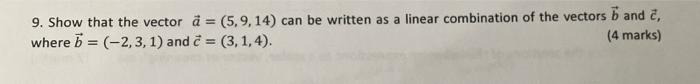# Question Solved1 Answer 9. Show that the vector a = (5,9,14) can be written as a linear combination of the vectors b and 2, (4 marks) where b = (-2, 3, 1) and = (3, 1, 4). 9. Show that the vector a = (5,9,14) can be written as a linear combination of the vectors b and 2, (4 marks) where b = (-2, 3, 1) and = (3, 1, 4).Transcribed Image Text: 9. Show that the vector a = (5,9,14) can be written as a linear combination of the vectors b and 2, (4 marks) where b = (-2, 3, 1) and = (3, 1, 4).
More
Transcribed Image Text: 9. Show that the vector a = (5,9,14) can be written as a linear combination of the vectors b and 2, (4 marks) where b = (-2, 3, 1) and = (3, 1, 4).